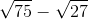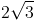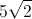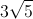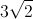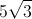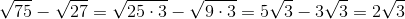# High School Math : Using Radicals with Elementary Operations

## Example Questions

### Example Question #1 : Using Radicals With Elementary Operations

What is the value of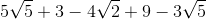?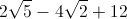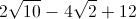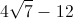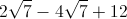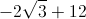Explanation:

When combining terms involving radicals, we can only combine the ones that have the same radical.  For this problem, that means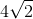has to stay on its own while we can combine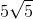and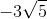into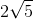.  The simple integers can be combined too, giving us our answer with three seperate terms.

### Example Question #2 : Using Radicals With Elementary Operations

Simplify the expression:.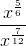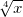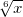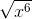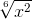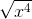Explanation:

Exponents in the denominator can be subtracted from exponents in the numerator.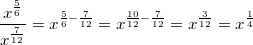Recall that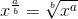.

Therefore,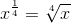.

### Example Question #3 : Using Radicals With Elementary Operations

Simplify: